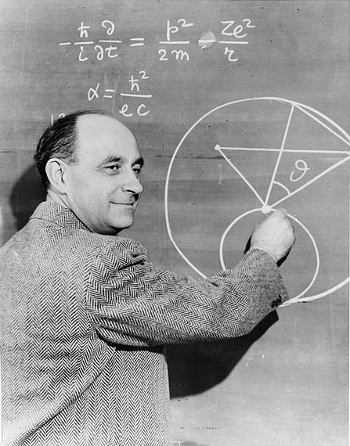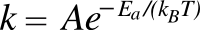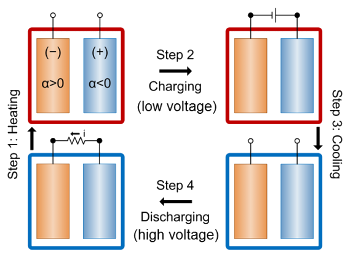### Thermogalvanic Cell

June 25, 2014

Rules of thumb are nice to have when no other data are available. The first such rule I learned is, "a pint's a pound the world round." If you insist on using the English system of units, as we do in the United States, this means that a gallon of water weighs about eight pounds, as does a gallon of milk, and a gallon of nearly every other liquid.

The actual values are 8.34 lb./gal. for water and 8.6 lb./gal. for whole milk. Gasoline is a lightweight, weighing in at just 6.1 lb./gal. Pure octane has a density of 5.84 lb./gal, while ethanol has a density of 6.58 lb./gal.

Another rule of thumb that I learned from a metallurgy professor is that the density of everything is five. That's because the average density of the Earth is 5.5, since it's a composite of crustal rock (density about 2.5), and the density of iron (about 7.87) comprising its core.

I wrote about other scientific rules of thumb in an earlier article (Rules of Thumb, September 5, 2008). These include the Dulong and Petit Law, that the specific heat of a material is always three times the gas constant (R) divided by the molar mass, and the Wiedemann-Franz Law, that the ratio of the thermal conductivity to the electrical conductivity of a metal is proportional to the temperature.

It's not surprising that there's a web site, rulesofthumb.org, devoted to this topic. This site has more than five thousand such rules, just a small fraction of which involve science and technology. One of my favorites there is Rule 1966, "The shorter the life of an elementary particle, the more it costs to make." Also entertaining is Rule 1686, "For mathematics professors, each published math paper is worth \$10,000 in salary."

Once you have such basic data, it's time for calculation. As I wrote in "Estimation" (December 21, 2011), Nobel physics laureate, Enrico Fermi was a master at estimating nearly anything, including the number of piano tuners in Chicago (in the days when many people still had mechanical pianos in their homes). His back-of-the-envelope calculations would infer the desired quantity by a very long chain of estimated values using simple models. There was a book, "Guesstimation: Solving the World's Problems on the Back of a Cocktail Napkin," published on this topic in 2008.Enrico Fermi at a blackboard.

The expression for the fine structure constant, α, is wrong. Fermi's smile might indicate an intentional joke.

Back-of-the-envelope calculations based on imperfect information are called "Fermi problems" in his honor.

(Smithsonian Institution photograph, via Wikimedia Commons)

All this is preface to an important chemist's rule of thumb that the rate of most reactions doubles every ten degrees Celsius. This rule is a consequence of the Arrhenius equation, which expresses the reaction rate k in terms of an activation energy Ea and absolute temperature T; viz.,Where R is the gas constant. This could be called the "chemist's" version of the equation, especially when the gas constant is expressed in calories as 1.9872 cal/K/mol. The "physicist's" version of the equation, shown below, uses the Boltzmann constant kB, instead. The units for the activation energy are different between these equations.Activation energies vary widely, but a reasonable value is 12.5 kcal. Plugging this into the Arrhenius equation gives a rate change of 1.96 when going from 300 K to 310 K. This large temperature dependence of reaction rate allows us to speed exothermic reactions by heating, or slow them by cooling. Changing the reaction rate also changes the equilibrium mixture of products and reactants.

Scientists from the Department of Materials Science and Engineering, Stanford University (Stanford, California), the Department of Mechanical Engineering, Massachusetts Institute of Technology (Cambridge, Massachusetts), and the Stanford Institute for Materials and Energy Sciences, SLAC National Accelerator Laboratory (Menlo Park, California) have demonstrated that such thermochemical effects can be used for thermal energy-harvesting at small differential temperatures. Their thermogalvanic cell is described in a recent issue of Nature Communications.[2-3]

The operation of such a thermogalvanic cell for energy-harvesting is shown in the figure. An electrochemical cell is heated so that its voltage becomes lower. It is then charged at a higher temperature, using a low voltage. After the cell is cooled, its voltage becomes higher, and it's discharged at this lower temperature at the high voltage. The heat energy is harvested from the voltage difference between the high and low temperature states.Energy-harvesting cycle of a thermogalvanic cell.

(MIT illustration.)
[3)]

The thermogalvanic cell is proposed for generating electricity through energy harvesting of the waste heat available from many processes. This heat is generally available only at a temperature just a hundred degrees Celsius higher than the Environment. Energy -harvesting of such heat sources is presently done by low efficiency thermoelectric devices. MIT's Gang Chen, an author of the study, explains that the concept goes back to the 1950s, but materials at that time could not make a reasonably effective device.

The electrochemical system is based on a copper hexacyanoferrate cathode and a Cu/Cu2+ anode. The device showed an energy conversion efficiency of 5.7% in cycling between 10°C and 60°C.[2-3] Says Yuan Yang, an author of the study and a postdoctoral associate in MIT's Mechanical Engineering Department, "One-third of all energy consumption in the United States ends up as low-grade heat."Thermodynamic cycle for a thermogalvanic cell. The cycle is based on the defining equation for the Gibbs Free Energy G; that is, G = H - TS, where H is the enthalpy, and S is the entropy. The theoretical amount of energy that can be harvested is the area defined by the cycle loop. (Drawing by the author using Inkscape.)

This method of thermal energy conversion has several present problems, the first of which is that it doesn't operate against a temperature gradient as thermoelectrics do; rather, the entire device needs to be heated and cooled in a cycle. Another problem, shared with all battery systems, is its low power density. Then there's the speed of charging and discharging.

Funding for this research came from the US Department of Energy, the US Air Force, and the National Research Foundation of Korea.

### References:

Linked Keywords: Rule of thumb; data; A pint's a pound the world round; English system of units; United States; gallon; water; pound; milk; liquid; whole milk; gasoline; octane; ethanol; metallurgy; professor; density; Earth; crust; rock; iron; core; science; scientific; Dulong and Petit Law; specific heat; material; gas constant; molar mass; Wiedemann-Franz Law; ratio; thermal conductivity; electrical conductivity; metal; proportional; temperature; web site; rulesofthumb.org; technology; Rule 1966; elementary particle; Rule 1686; mathematics; scientific literature; publish; salary; calculation; Nobel Prize in Physics; Nobel laureate; Enrico Fermi; piano tuning; piano tuner; Chicago; mechanical piano; home; back-of-the-envelope calculation; scientific modelling; book; blackboard; fine structure constant; smile; joke; Fermi problem; Smithsonian Institution; photograph; Wikimedia Commons; chemist; reaction rate; chemical reaction; Celsius; Arrhenius equation; activation energy; absolute temperature; calorie; physicist; Boltzmann constant; kcal; Kelvin; K; exothermic reaction; heating; cooling; equilibrium; product; reactant; Department of Materials Science and Engineering; Stanford University (Stanford, California); Department of Mechanical Engineering; Massachusetts Institute of Technology (Cambridge, Massachusetts); Stanford Institute for Materials and Energy Sciences; SLAC National Accelerator Laboratory (Menlo Park, California); thermochemistry; thermochemical; thermal energy-harvesting; Nature Communications; electrochemical cell; voltage; battery charger; battery; discharge; heat energy; electricity generation; generating electricity; waste heat; environment; energy conversion efficiency; thermoelectric effect; Gang Chen; author; copper; ferrocyanide; hexacyanoferrate; cathode; anode; Yuan Yang; postdoctoral associate; Mechanical Engineering Department; United States; Gibbs Free Energy; enthalpy; entropy; theory; theoretical; energy; energy harvesting; area; Inkscape; temperature gradient; power density; US Department of Energy; US Air Force; National Research Foundation of Korea; Lawrence Weinstein and John A. Adam, "Guesstimation: Solving the World's Problems on the Back of a Cocktail Napkin, Princeton University Press, April 21, 2008.